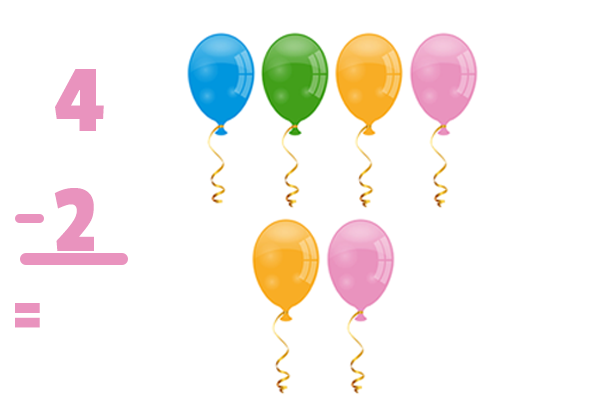# Subtraction Under 10

Description: Subtract numbers under 10.

Tags: Kindergarten, CCSS.MATH.CONTENT.K.OA.A.1, Subtraction

Question 1 (Multiple Choice, 10 points)

What is 5-2=?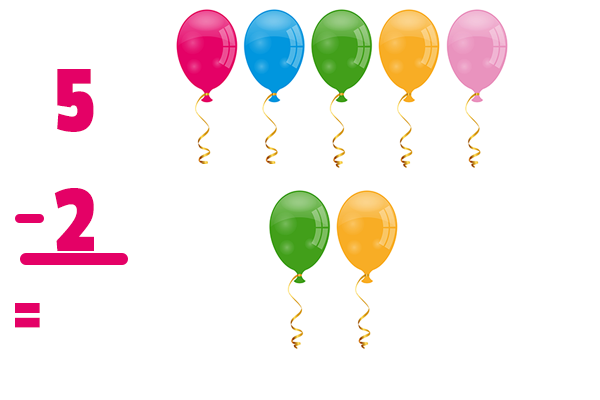Question 2 (Multiple Choice, 10 points)

What is 7-3=?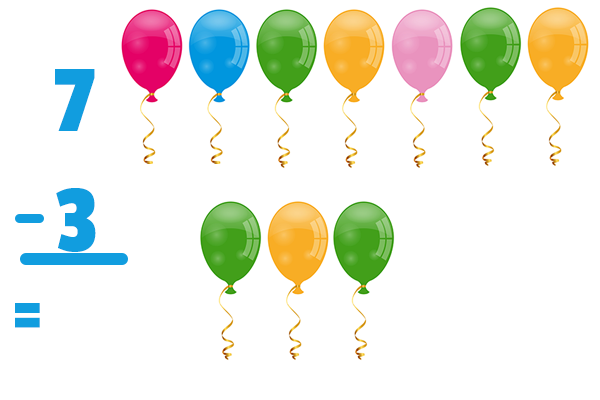Question 3 (Short Answer, 10 points)

What is 9-6=?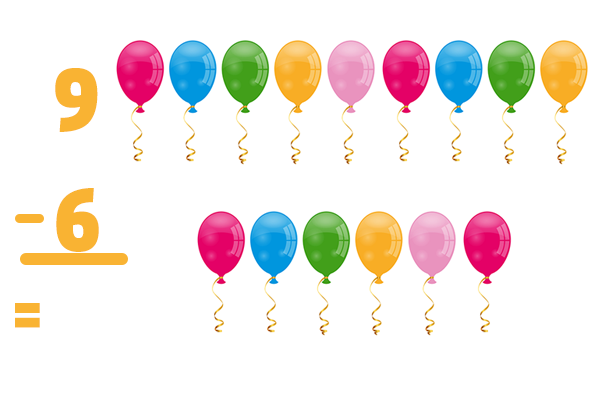Question 4 (Short Answer, 10 points)

What is 5-3=?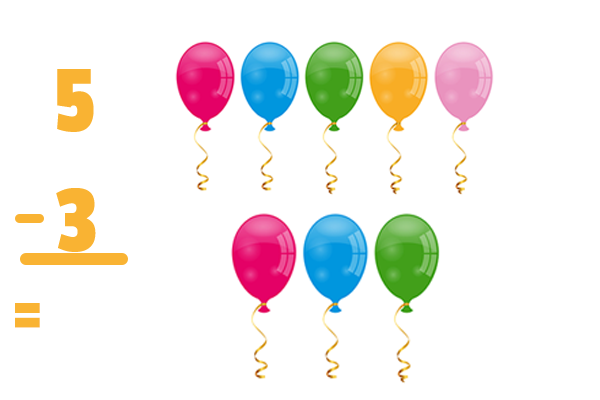Question 5 (Short Answer, 10 points)

What is 4-2=?